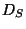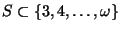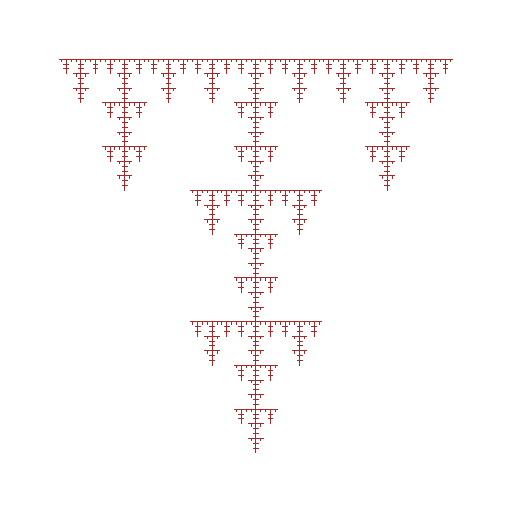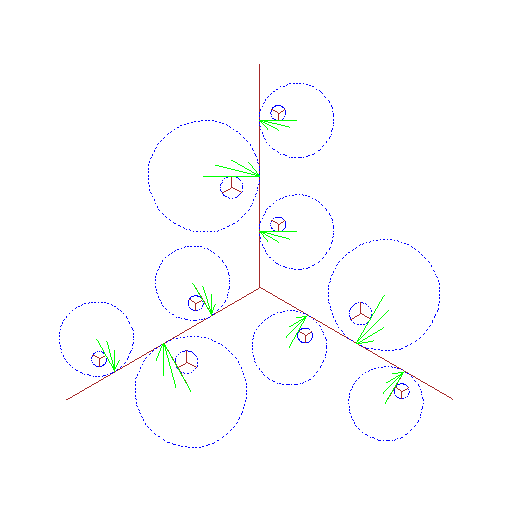Next: Monotone equivalence and monotone Up: Dendrites Previous: The dendrite

## Self-homeomorphic dendrites

Below we present some examples of dendrites that illustrate relations between variants of self-homeomorphic properties.

1. There is a strongly pointwise self-homeomorphic dendrite which is not of the formfor some, see [Charatonik W.J. et al. 1994, Example 6.11, p. 232, and Fig. 4, p. 233]. Note that, according to Property 1 in 1.3.8 each dendriteis strongly pointwise self-homeomorphic. This example shows that the opposite implication does not hold. Some additional properties of the example are shown in [Pyrih 1999b, Example 2.1, p. 152]. See Figure A.2. There is a strongly self-homeomorphic and pointwise self-homeomorphic dendrite which is not strongly pointwise self-homeomorphic, see [Charatonik W.J. et al. 1994, Example 6.12, p. 233].

3. There is a strongly self-homeomorphic dendrite which is not pointwise self-homeomorphic, see [Pyrih 1999a, Example 2.1, p. 572]. Dendrites having this property are studied in [Charatonik et al. XXXXc].

See Figure B.Here you can find source files of this example.

Here you can check the table of properties of individual continua.

Here you can read Notes or write to Notes ies of individual continua.Next: Monotone equivalence and monotone Up: Dendrites Previous: The dendrite
Janusz J. Charatonik, Pawel Krupski and Pavel Pyrih
2001-11-30